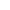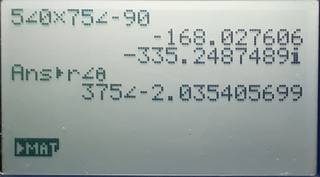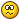# Electrical calculations with vectors

vectors electrical calculationsBest Answer pan.gejt , 12 September 2021 - 10:55 AM

Try your calculations based on picture

∠(r,θ) calculations for electric circuits

https://edu.casio.co...s/cg/cp330plus/

According to the picture the input is in form ∠(r,θ) instead of input as a vector.

Go to the full post3 replies to this topic

### #1marko

marko

Newbie

•• Members
•• 2 posts

• Calculators:

Posted 12 September 2021 - 08:53 AM

Hello. I am an electrical engineering student and we have a lot of calculations with AC circuits.

Im am trying to figure out how to use the calculator for the following calculations.

Calculator in use: ClassPad II fx-CP400

Here are the examples:I was able to calculate the ones where I just add them together, like this:But when I try dividing or multiplying, I get this:Always the same error, Invalid Dimension.

Sometimes when I have to calculate the impedance of a circuit, I would also need to somehow enter the following into the calculator:But here I also get the error: Invalid Dimension.

I am very close to just throwing this calculator away and getting an TI, where I know calculations like these are very easy and do not require the "[" and "]" for example.

Any help would be appreciated. I've been searching the internet for a long time now and for some reason can not figure it out.

### #2pan.gejt

pan.gejt

Casio Freak

•• Members
•• 249 posts
• Gender:Male
• Location:CZ

• Calculators:
.

Posted 12 September 2021 - 10:55 AM   Best Answer

Try your calculations based on picture

∠(r,θ) calculations for electric circuits

https://edu.casio.co...s/cg/cp330plus/

According to the picture the input is in form ∠(r,θ) instead of input as a vector.

• marko likes this

### #3Hlib2

Hlib2

Casio Freak

•• Members
•• 139 posts
• Gender:Male
• Location:Ukraine
• Interests:industrial electronics,
graphing calculators

• Calculators:
fx-9860GII-2,
cfx-9850GC+,
fx-9750G+, graph_100+,
fx-9750GII, fx-991DE_X,
ti-83+_SE, ti-84+, ti-85,
ti-89_Titanium,
ti-voyage200.

Posted 12 September 2021 - 01:10 PM

In your original example, degrees are specified as arguments, but it is clear from the calculation result that your calculator is set to Rad mode. This is not significant, but it makes confusion.
I have tested multiplication and division from the above examples on afx-2.0+ and on fx-9750GII. There were no any problems.I don`t have an fx-CP400, but I can assume the following: your calculator in the above image, apparently, operates with the type of variables Mat or Vct. Multiplying a string by a string causes the message Invalid Dimension. Does this calculator have a different syntax for such calculations?
This is a formal approach to the problem. It seems to me that in electrical engineering, when calculating with vectors, the commands DotP (dot product) or CrsP (cross product) should be also used.
• marko likes this

### #4marko

marko

Newbie

•• Members
•• 2 posts

• Calculators:

Posted 12 September 2021 - 02:52 PM

Big thanks to both of you!

@pan.gejt

All I indeed had to is what was in the link you sent:

```∠(5,θ) * ∠(75,-90)
```

gives me exactly the results I need.

@Hlib2

My calculator was in RAD mode and first I kept getting weird results with pan.gejts suggestion, but changing to DEG fixed it.

I am not familiar with DotP (dot product) or CrsP. I will look into those as well, but for now I am very happy with being able to do these calculations.

It just means now that I must do my homework.

### Also tagged with one or more of these keywords: vectors, electrical calculations

#### 0 user(s) are reading this topic

0 members, 0 guests, 0 anonymous users